﻿ 現金流量

# 現金流量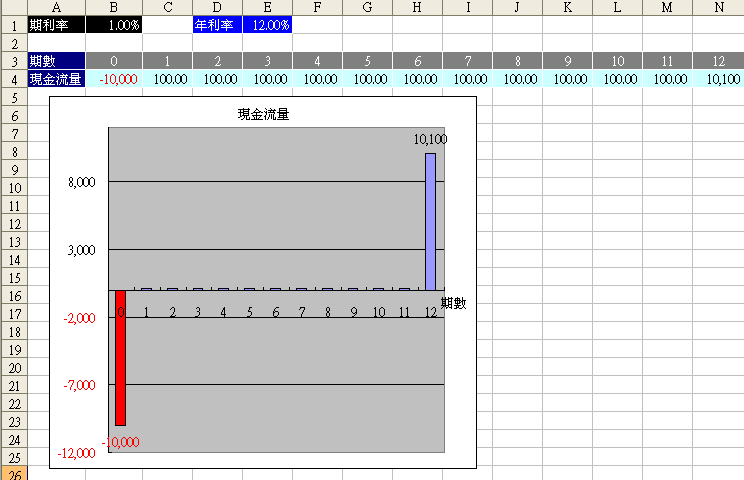# 現金流量有何用途

PV = 100/(1+rate)1 + 100/(1+rate)2+ 100/(1+rate)3+ 100/(1+rate)4+ 100/(1+rate)5+ 100/(1+rate)6+ 100/(1+rate)7+ 100/(1+rate)8+ 100/(1+rate)9+ 100/(1+rate)10+ 100/(1+rate)11+ 10100/(1+rate)12

## 以Excel IRR函數計算

rate =IRR(B4:N4) = 1.00%

= 100/(1+1%)^1 + 100/(1+1%)^2 + 100/(1+1%)^3 + 100/(1+1%)^4 + 100/(1+1%)^5 + 100/(1+1%)^6+ 100/(1+1%)^7+ 100/(1+1%)^8+ 100/(1+1%)^9+ 100/(1+1%)^10+ 100/(1+1%)^11+ 10100/(1+1%)^12
=10,000

=NPV(1%,100,100,100,100,100,100,100,100,100,100,100,10100)
=10,000

## 現金流量反推報酬率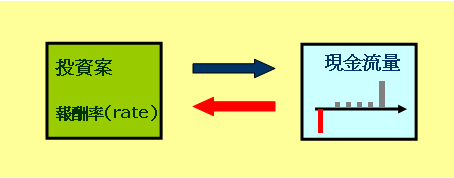# 淨現金流量

## 貸款案例

=-1000000 + 6000+12000 = -982,000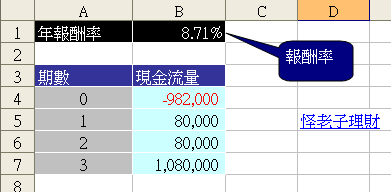=IRR({-982000,80000,80000,1080000})=IRR(B4:B7)
=8.71%

## 房屋出租例子

=pmt(2.5%/12,240,6000000)
=-31,794

=25000-31794
=-6,794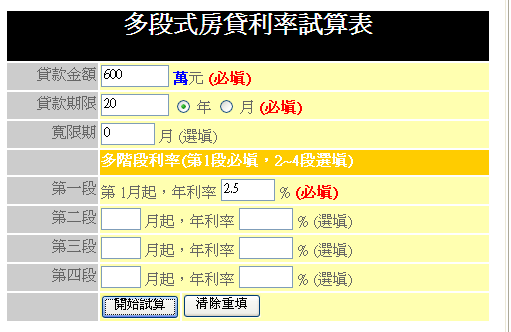=8000000-3372675+25000-31794
=4,620,531

 期初 -2,000,000 第1期 -6,794 第2期 -6,794 第3期 -6,794 ..... .... 第119期 -6,794 第120期 4,620,531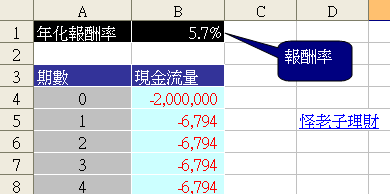=RATE(120,-6794,-2000000,4620531)*12
=5.7%

# 相關文章

EXCEL投資理財應用

﻿
﻿

■ 本網站內容儘可能精確完整，但不保證無誤。若做為投資依據，風險請自行斟酌 ，本網站不負賠償之責任。
■ 網站所有資料均為版權所有，非經書面允許請勿轉載或使用。